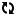# Grafica

Pictures
Title### Grafica - DXT 4109

Questo programma consente la rappresentazione grafica
dei dati inseriti, sotto forma di diagrammi a barre e a torta.
Oltre a ciò potete disegnare sul video funzioni a due e tre
dimensioni. I valori della funzione e la relativa derivata
prima in vari punti del grafico possono essere evidenziati
per mezzo del Joystick.

Developer: Original developer of the US release (CX4109): Lane Winner (uncredited) / Howard D. Siebenrock
According to source code (REM at line 0) (see below), it’s exactly the same rev numbers than the german release “Graph It aka Graph IT II aka Graph IT Enhanced – TXG 9517”

Crédits :
Loading screen : 0 REM ENHANCED BY HOWARD SIEBENROCK REV 1.2 10-30-81
Bar : 0 REM GRAPH IT BAR-PIE REV 0.1 7-5-80 ENHANCED BY HOWARD SIEBENROCK REV. 1.3 11.13.81
Graph XY :  0 REM GRAPH.XY ENHANCMENTS BY HOWARD SIEBENROCK REV 1.3 11-13-81 (Yes, including the typo at « ENHANCMENTS » ! 😉 – same typo in the german release)
Polar : 0 REM GRAPH POL:REV 0.3 9-25-80 ENHANCED BY HOWARD SIEBENROCK VES. 1.3 11-14-81
3D : 0 REM 3D REV. 0.1 8-15-80 ENHANCED BY HOWARD SIEBENROCK REV 1.3 11-15-81

Date: 1984 according to cover, 1981 according to source code, no date on the booklet.
Distributed by: ATARI International (Italy) Inc. V.le della Liberazione, 18 – 20124 Milano
Notes: Translation and enhancement of « Graph IT » – CX4109 – USA.
How to run:  Load .PRO in your emulator (Altirra), boot without « Option » key. Enable Disk SIO Patch and Fast FP Math if you don’t want to wait.
Other translations :
USA: « Enhancements to Graph It » – APX 20074 – 1982
Germany: Graph It aka Graph IT II aka Graph IT Enhanced – TXG 9517
Atariwiki reports that another release was also made for Germany with a different source code of « GRAPH IT BAR-PIE » in 1982.

Rendering comparison (“Grafica 3D”) :

1 – Heart
Atari Formula : 5+​(‑sqr(​1-​x^​2-​(y-​abs(​x))^​2))*​cos(​30*​(1-​x^​2-​(y-​abs(​x))^​2))
Google Formula : 5 + (-sqrt(1-x^2-(y-abs(x))^2))*cos(30*((1-x^2-(y-abs(x))^2))), x is from -1 to 1, y is from -1 to 1.5, z is from 1 to 62 – Water drop
Atari Formula : sqr(x*x+y*y)+3*cos(sqr(x*x+y*y))+5
Google Formula : sqrt(x*x+y*y)+3*cos(sqrt(x*x+y*y))+5 from -20 to 203 – Egg
Atari Formula : 1.2+(sqr(1-(sqr(x^2+y^2))^2)+1–x^2-y^2)*(sin(10*(x*3+y/5+7))+1/4)
Google Formula : 1.2+(sqrt(1-(sqrt(x^2+y^2))^2)+1–x^2-y^2)*(sin(10*(x*3+y/5+7))+1/4) from -1.6 to 1.64 – Wheel

Atari Formula : (0.4^2-(0.6-(x^2+y^2)^0.5)^2)^0.55 – Pyramid

Atari Formula : 1-abs(x+y)-abs(y-x)6 – Waves

Atari Formula : x*sin(-(x*x)-(y*y))
Google Formula : x*sin(-(x*x)-(y*y)) from -5 to 5Media Type Country Doc
A
A

A

Rank it !
 Gameplay 3 Graphics 3 Sound 3 Ergonomy 3 Interest 3 Rarity 3 MoyenneAucun Commentaire »